Friday, February 28, 2020

What Do You Call a Line That Crosses Two Other Lines?

(Click on the comic if you can't see the full image.)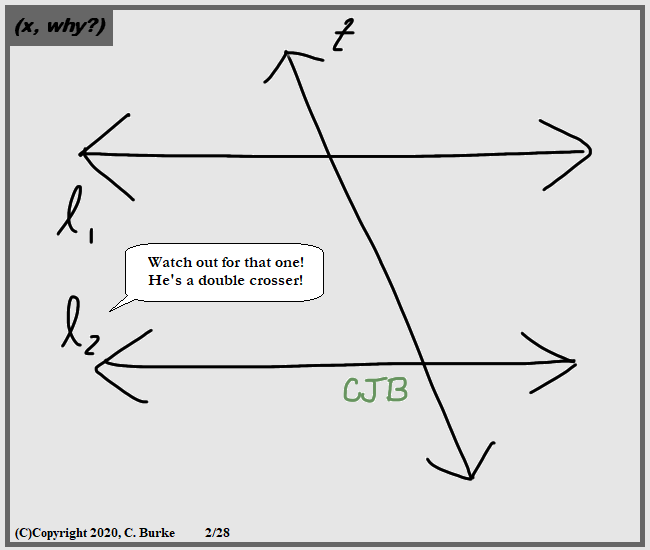(C)Copyright 2020, C. Burke. "AnthroNumerics" is a trademark of Christopher J. Burke and (x, why?).

And sometimes transversals can even be triple crossers! So watch out!

Come back often for more funny math and geeky comics.Monday, February 24, 2020

You Snooze, You Lose, Part II

(Click on the comic if you can't see the full image.)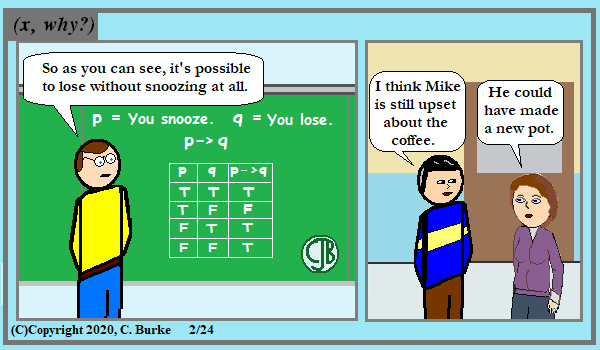(C)Copyright 2020, C. Burke. "AnthroNumerics" is a trademark of Christopher J. Burke and (x, why?).

It is not the case that if one does lose, then one did snooze.

C'mon! The previous comic was screaming out for a Truth Table!

Come back often for more funny math and geeky comics.Saturday, February 22, 2020

You Snooze, You Lose

(Click on the comic if you can't see the full image.)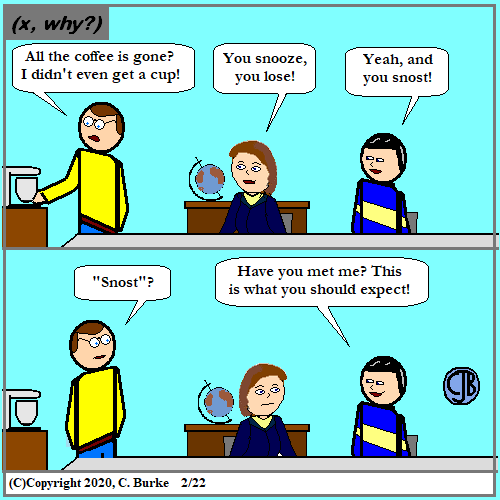(C)Copyright 2020, C. Burke. "AnthroNumerics" is a trademark of Christopher J. Burke and (x, why?).

Well, you lost, right?

Come back often for more funny math and geeky comics.Wednesday, February 19, 2020

Just One Thing

(Click on the comic if you can't see the full image.)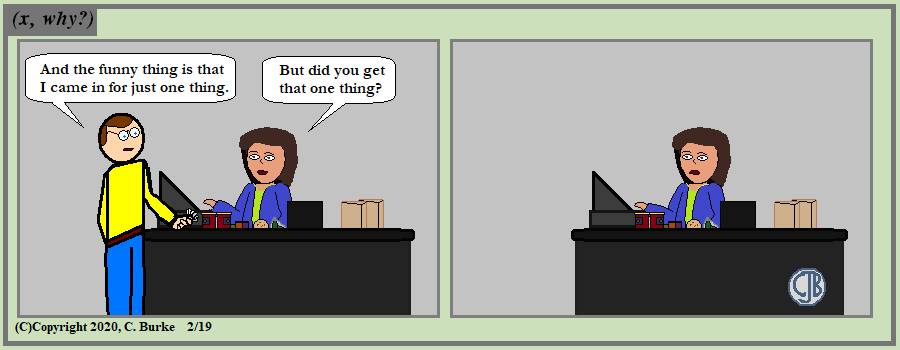(C)Copyright 2020, C. Burke. "AnthroNumerics" is a trademark of Christopher J. Burke and (x, why?).

Based on semi-autobiographical events.

Come back often for more funny math and geeky comics.Tuesday, February 18, 2020

January 2020 Geometry Regents Parts 3 & 4

The following are some of the multiple questions from the January 2020 New York State Geometry Regents exam.

January 2020 Geometry, Part III

Each correct answer is worth up to 4 credits. Partial credit is available. Work must be shown. Correct answers without work receive only 1 point.

32. Quadrilateral NATS has coordinates N(-4,-3), A(1,2), T(8,1), and S(3,-4).
Prove quadrilateral NATS is a rhombus.
[The use of the set of axes below is optional.]

Shortest method: If the diagonals are perpendicular, the quadrilateral is a rhombus.
Other method: All four sides have the same length -- distance formula four times.
Other method: Opposite sides are parallel (same slope) and two consecutive sides have the same length -- distance formula two times.

Method 1:
Show that NT is perpendicular to AS:
NT: (1 - -3) / (8 - -4) = 4 / 12 = 1/3
AS: (-4 - 2) / (3 - 1) = -6 / 2 = -3
Diagonal NT is perpendicular to AS, therefore the quadrilateral is a rhombus.
(You MUST have a concluding statement!)

Method 2:
NA = SQRT( (1 - -4)2 + (2 - -3)2) = SQRT(25 + 25) = SQRT(50)
AT = SQRT( (8 - 1)2 + (1 - 2)2) = SQRT(49 + 1) = SQRT(50)
TS = SQRT( (3 - 8)2 + (-4 - 1)2) = SQRT(25 + 25) = SQRT(50)
SN = SQRT( (-4 - 3)2 + (-3 - -4)2) = SQRT(49 + 1) = SQRT(50)
All four sides are congruent, therefore the quadrilateral is a rhombus.

Method 3:
NA = SQRT( (1 - -4)2 + (2 - -3)2) = SQRT(25 + 25) = SQRT(50)
AT = SQRT( (8 - 1)2 + (1 - 2)2) = SQRT(49 + 1) = SQRT(50)
(Note that the math involved in finding the slope is the same that was done in Method 2)
Slope of AT: -1/7
Slope of SN: -1/7
Slope of NA: 5/5 = 1
Slope of TS: 5/5 = 1
Opposite sides are parallel and consecutive sides are congruent, therefore the quadrilateral is a rhombus.

33. David has just finished building his treehouse and still needs to buy a ladder to be attached to the ledge of the treehouse and anchored at a point on the ground, as modeled below. David is standing 1.3 meters from the stilt supporting the treehouse. This is the point on the ground where he has decided to anchor the ladder. The angle of elevation from his eye level to the bottom of the treehouse is 56 degrees. David’s eye level is 1.5 meters above the ground.

Determine and state the minimum length of a ladder, to the nearest tenth of a meter, that David will need to buy for his treehouse.

You need to find the height of the stilt, but you don't have an angle from the ground. Instead you have the angle from David's eye level. So you have to find that height of that portion of the stilt and then ADD 1.5 meters, which is the height of David's eye. That will give you the height of the stilt.

One you have the height of the stilt and the distance along the ground, you have the two legs of a right triangle. The ladder will be the hypotenuse. Use the Pythagorean Theorem, and round to the nearest tenth of an inch.

tan 56 = x / 1.3
x = 1.3 tan 56 = 1.927329. Do NOT round in the middle of the problem.
1.927329 + 1.5 = 3.427329 This is the Stilt height.
a2 + b2 = c2
1.32 + 3.4273292 = 13.436584
c = SQRT(13.436584) = 3.665 = 3.7 meters.

Note: you don't need to keep 7 or 8 decimal places, but if they are already in the calculator's memory, leave them there. If you round, always keep 2 or 3 decimal digits or you will have rounding errors in your final answer.

34. A manufacturer is designing a new container for their chocolate-covered almonds. Their original container was a cylinder with a height of 18 cm and a diameter of 14 cm. The new container can be modeled by a rectangular prism with a square base and will contain the same amount of chocolate-covered almonds.

If the new container’s height is 16 cm, determine and state, to the nearest tenth of a centimeter, the side length of the new container if both containers contain the same amount of almonds.
A store owner who sells the chocolate-covered almonds displays them on a shelf whose dimensions are 80 cm long and 60 cm wide. The shelf can only hold one layer of new containers when each new container sits on its square base. Determine and state the maximum number of new containers the store owner can fit on the shelf

The first part of the problem wants you to compare Volumes. This is a semi-realistic problem. It's realistic because manufacturers change packaging for business reasons. It's less realistic because the Volume itself doesn't take empty space between almonds and the sides of the container into account. These could be different in different-shaped packaging. But this isn't a marketing exam.
Volume = Area of the Base times the Height.
The Area of the cylinder base is (pi)(r)2. The Area of the square prism base is s2.
Use the pi key on your calculator. And remember to cut the diameter in half to get the radius.

Volume of the cylinder: (3.141592)(7)2(18) = 2770.8841...
Volume of prism: s2 * 16 = 2770.8841
s2 = 173.18
s = 13.15... = 13.2
Area of the base = (6)(10) + (1/2)(6)(2.65) = 67.95
Volume = Area * height = 67.95 * 6.5 = 441.675 = 442 cubic feet

The second part asks you to determine the number of boxes that can fit on the shelf. Do NOT compare Areas because the boxes cannot be split apart to fit into leftover spaces.
Find the maximum number of boxes that can fit on the shelf from front to back, and from side to side. Then multiply those numbers.

80 / 13.2 = 6.06... = 6
60 / 13.2 = 4.54... = 4, round down. There is no space to round up.
6 * 4 = 24 boxes can be displayed on one shelf.

Part IV

A correct answer is worth up to 6 credits. Partial credit is available.

35. In quadrilateral ABCD, E and F are points on BC and AD, respectively, and BGD and EGF are drawn such that ∠ABG = ∠CDG, AB = CD, and CE = AF.

Prove: FG = EG

You can prove that FG = EG if you can prove that triangles BGE = DGF.
First, mark off what you know, so you can figure out how to proceed.

The image *looks* like a parallelogram, but we have to prove that it is a parallelogram (which we can).
Once we know that we'll know that BC and AD are both parallel and congruent. This will make BE and DF congruent through the Subtraction property. Also, BD and EF create pairs of vertical angles, which of congruent, but this needs to be stated as well.

 Statement Reason 1. ∠ABG = ∠CDG, AB = CD, and CE = AF. Given 2. AB || CD If the Alternate Interior Angles are congruent, the lines are parallel 3. ABCD is a parallelogram If an opposite pair of sides of a quadrilateral are both congruent and parallel, the quadrilateral is a parallelogram. 4. BC = AD Opposite sides of a parallelogram are congruent. 5. BE = DF Subtraction property. 6. BC || AD Opposite sides of a parallelogram are parallel. 7. ∠EBG = ∠ADG Alternate Interior angles. 8. ∠BGE = ∠DGF Vertical angles. 9. Triangle BGE = Triangle DGF AAS 10. FG = EG CPCTC (Corresponding Parts of Congruent Triangles are Congruent)

You could also have shown that triangle ABD and CDB were congruent. In that case, CPCTC would give you angles CBG and FDG, and AD = CB. Then you could use the Subtraction property to get the smaller triangles.

End of Exam

How did you do?
Comments and corrections welcome. (I get many of the latter!)

I also write Fiction!

Check out In A Flash 2020, by Christopher J. Burke for 20 great flash fiction stories, perfectly sized for your train rides.
Available in softcover or ebook at Amazon.

If you enjoy it, please consider leaving a rating or review on Amazon or on Good Reads.

Thank you.Monday, February 17, 2020

(x, why?) Mini: Canceling

(Click on the comic if you can't see the full image.)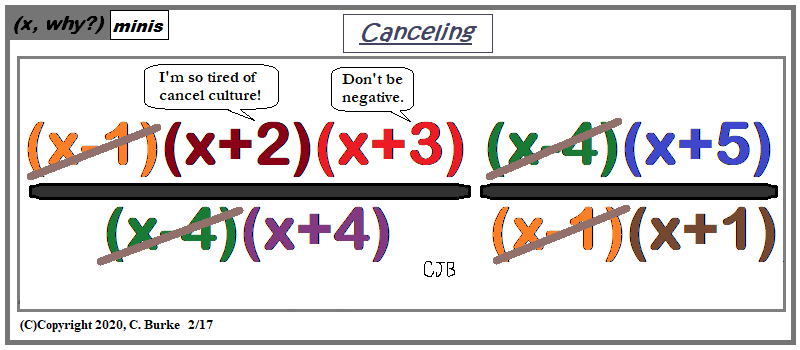(C)Copyright 2020, C. Burke. "AnthroNumerics" is a trademark of Christopher J. Burke and (x, why?).

Eliminate. Terminate. Totally, completely Annihilate.

Someone obviously used the Difference of Square rule in this example.

Come back often for more funny math and geeky comics.Saturday, February 15, 2020

January 2020 Geometry Regents, Part 2

The following are some of the multiple questions from the recent January 2020 New York State Common Core Geometry Regents exam.

January 2020 Geometry, Part II

Each correct answer is worth up to 2 credits. Partial credit can be given. Work must be shown or explained.

25. In the diagram below, right triangle PQR is transformed by a sequence of rigid motions that maps it onto right triangle NML.

Write a set of three congruency statements that would show ASA congruency for these triangles.

Pick two pairs of corresponding angles and a pair of corresponding sides.
Note: Use the Congruency symbol, not the Equal sign. (I don't have the option on this blog.)
<Q = <M ; <R = <L ; QR = ML ;

26. Diego needs to install a support beam to hold up his new birdhouse, as modeled below. The base of the birdhouse is 24 1/2 inches long. The support beam will form an angle of 38° with the vertical post. Determine and state the approximate length of the support beam, x, to the nearest inch.

We don't care about the birdhouse. We only care about the right triangle under it.
We know the side opposite the angle and the hypotenuse, so we need to use Sine.

Sin 38° = 24.5 / x
x = 24.5 / Sin 38° = 39.79 = 40 inches.

27. A rectangular tabletop will be made of maple wood that weighs 43 pounds per cubic foot. The tabletop will have a length of eight feet, a width of three feet, and a thickness of one inch.
Determine and state the weight of the tabletop, in pounds.

To find the weight, you first need to find the Volume in cubic feet, and then multiply it by the weight of the wood. You have to convert the inch into 1/12 feet.

V = l * w * h = (8) (3) (1/2) = 2
W = 43 * 2 = 86 pounds

28. In the diagram below of circle O, secant ABC and tangent AD are drawn.

If CA = 12.5 and CB = 4.5, determine and state the length of DA.

DA = 10

29. Given ___ MT below, use a compass and straightedge to construct a 45° angle whose vertex is at point M. [Leave all construction marks.]

The easiest way I would think to do this is to create a 90-45-45 triangle, which M as one of the vertices. You construct a perpendicular bisector of MT, and then draw an arc from the right angle that has a length equal to the base of the triangle.

Step one: From M, make arcs above and below the line that go at least halfway across.
Then, without changing the size of the compass, go to T and repeat the process. You should have marked off two Xs.
With a straightedge, draw a line between the center of those two Xs. That is a perpendicular bisector.
Let's give the right angle a name, call it "R". You don't have to do this, but it will help me explain the following step.

Step two: From R, open the compass to point M. Draw an arc up to the vertical line. Call that point "S". With a straightedge, draw MS. Label angle M 45°. (Note that angle MSR is also 45°.)

[IMAGE OMITTED]

30. In triangle XYZ shown below, medians XE, YF, and ZD intersect at C.
If CE = 5, YF = 21, and XZ = 15, determine and state the perimeter of triangle CFX.

The medians cut the sides of XYZ in half. The medians themselves intersect at the centroid which divides the median into a ratio of 2:1.

If CE = 5, that means that CX = 10 (and EX = 15).
If YF = 21, that means that CF = 7 (and YC = 14).
If XZ = 15, that FX = 7.5.
So the perimeter of CFX ix 10 + 7 + 7.5 = 24.5.

31. Determine and state an equation of the line perpendicular to the line 5x - 4y = 10 and passing through the point (5,12).

Short answer: Since it is in Standard form, switch the coefficients and change the sign to get a perpendicular line.
4x + 5y = C
4(5) + 5(12) = 20 + 60 = 80
4x + 5y = 80

The slope of 5x - 4x = 10 is -(5/-4) = 5/4
The perpendicular slope is the inverse reciprocal -4/5.
y - 12 = -4/5(x - 5)

Long way: put everything in slope-intercept form and slog it out:
5x - 4y = 10
-4y = -5x + 10
y = 5/4x - 10/4
Perpendicular line: y = -4/5 x + b
12 = -4/5 (5) + b
12 = -4 + b
16 = b
y = -4/5 x + 16

End of Part II

How did you do?

I also write Fiction!

Check out In A Flash 2020, by Christopher J. Burke for 20 great flash fiction stories, perfectly sized for your train rides.
Available in softcover or ebook at Amazon.

If you enjoy it, please consider leaving a rating or review on Amazon or on Good Reads.

Thank you.Friday, February 14, 2020

Happy Valentines Day 2020

(Click on the comic if you can't see the full image.)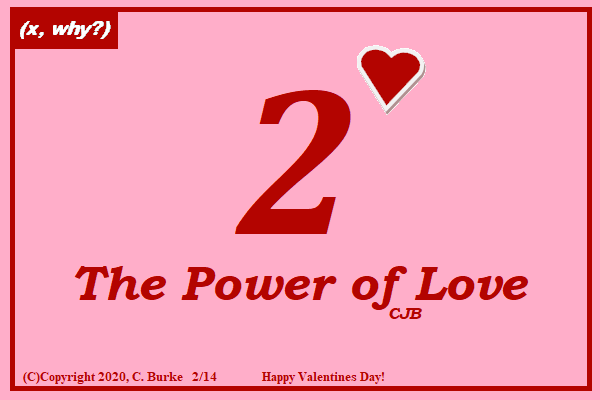(C)Copyright 2020, C. Burke. "AnthroNumerics" is a trademark of Christopher J. Burke and (x, why?).

Happy Valentines Day!
Or Happy St. Valentines Day, if you prefer.

I didn't know whether to make a Huey Lewis, Celine Dion, or Air Supply reference, so I just mentioned all three of them. That's the News, I'm sticking with it.

Come back often for more funny math and geeky comics.Thursday, February 13, 2020

Alternate Interior

(Click on the comic if you can't see the full image.)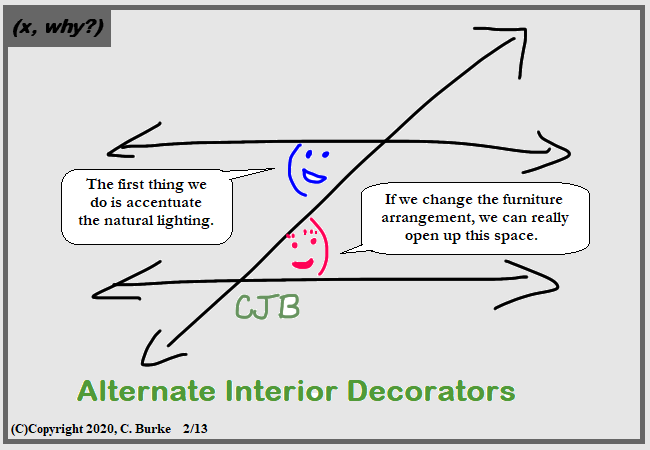(C)Copyright 2020, C. Burke. "AnthroNumerics" is a trademark of Christopher J. Burke and (x, why?).
Clear, crisp lines will define any space you design.

Come back often for more funny math and geeky comics.Wednesday, February 12, 2020

January 2020 Algebra 1 Regents Part 1 (multiple choice)

The following are some of the multiple questions from the recent January 2020 New York State Common Core Algebra I Regents exam.

Omitted images will be added soon.

January 2020 Algebra I, Part I

Each correct answer is worth up to 2 credits. No partial credit. Work need not be shown.

1. If f(x) = 2(3x) + 1, what is the value of f(2)?

f(2) = 2(32) + 1 = 2(9) + 1 = 18 + 1 = 19

2. A high school sponsored a badminton tournament. After each round, one-half of the players were eliminated. If there were 64 players at the start of the tournament, which equation models the number of players left after 3 rounds?

Answer: (1) y = 64(1 - .5)3
If you are eliminated half, then you are removing 50% or .5 of the teams. The number of teams is getting smaller, so eliminate the choices with "+". The exponent refers to the number of rounds.

This is an easy one to check: 64, 32, 16, 8. There are 8 teams after three rounds.
64(1 - .5)3 = 64(.5)3 = 64(.125) = 8

3. Given 7x + 2 > 58, which number is not in the solution set?

Note that for questions like this, when it is a linear inequality, not quadratic or something quirky, the answer is usually the smallest or the greatest value of the choices. You can check them first by substitution.
7(6) + 2 > 58
42 + 2 > 58
44 > 58
This is true. If you substitute 8, you will get exactly 58, which is equal, and the other two numbers will be greater.

7x + 2 > 58
7x > 56
x > 8

4. Which table could represent a function?

If a value repeats in the x column with different values in the y column, it cannot be a function. It will fail the vertical line test. One input cannot have multiple outputs in a function.

5. Which value of x make (x - 3) / 4 + 2 /3 = 17 / 12 true?

You can plug choices in. You can find a common denominator between 3 and 4 and add the fractions. Or you can multiply the entire equation by 12 to get rid of all the fractions.

Note: If this doesn't look right, I'll replace it with an image as soon as I can.

(12)[(x - 3) / 4 + 2 /3] = [17 / 12](12)
3(x - 3) + 4(2) = 17
3x - 9 + 8 = 17
3x - 1 = 17
3x = 18
x = 6

6. Which expression is equivalent to 18x2 - 50?

Answer: (3) 2(3x - 5)(3x + 5)
When you have a binomial like this, the first thing you check for is "Difference of Two Squares". Neither 18 nor 50 are perfect squares. However, if you factor them by 2, you get 2(9x2 - 25), and that binomial is the difference of two squares.
That means it can be factored into two conjugates, which will be the same two terms, one separated by a plus, and one separated by a minus: 2(3x - 5)(3x + 5).

7. The functions f(x) = x2 - 6x + 9 and g(x) = f(x) +k are graphed below.

Which value of k would result in the graph of g(x)?

Adding k to a function will translate the function up or down. Since the vertex of f(x) is at (3,0) and the vertex of g(x) is at (3,-2), the value of k must be -2.

8. The shaded boxes in the figure below represent a sequence.

If figure 1 represents the first term and this pattern continues, how many shaded blocks will be in figure 35?

The first term has 12 boxes shaded. Each term after that adds 4 more boxes.
To get from figure 1 to figure 35, you have to add four 34 times.
So Figure 35 will have 12 + (34)(4) = 148 boxes.

9. The zeros of the function f(x) = x3 - 9x2 are

There is no constant term in the function, so if x = 0, then f(x) = 0. Choice (1) is incorrect.
You can work backward from the choices to see that 9 is a solution and that 3 and -3 are not.
Or you can solve x3 - 9x2 = 0
x2(x - 9) = 0
x = 0 or x = 9

At no point is this a Difference of Squares problem.

10. A middle school conducted a survey of student to determine if they spent more of their time playing games or watching videos on their tablets. The results are shown in the table below.

Of the students who spent more time playing games on their tablets, approximately what percent were boys?

There were 138 boys and a total of 192 people in the Playing Games column. Turn that into a percent.
138 / 192 = 0.71875, or 72%

11. Which statement best describes the solutions of a two-variable equation?

Answer: (1) The ordered pairs must lie on the graphed equation.
This is by design. You graph the line to find the solutions.
If the points are "near", they are "almost" correct, but still not correct.
There will be times when you want to find a point on the x-axis or y-axis, but those are not the only solutions to an equation.

12. The expression x2 - 10x + 24 is equivalent to

Answer: (4) (x - 6)(x - 4)
This is a popular question because either of the signs could be changed and you would still have the same four choices (only different answers).
The + 24 tells us the signs will be the same. The - 10x tells us that both of those signs will be negative.
(-6)(-4) = + 24. (-6x) + (-4x) = -10x

13. Which statement is true about the functions f(x) and g(x), given below?

Answer: (2) f(x) and g(x) have the same y-intercept
If you read all the choices first, this one would have been the easiest to check, and it was the correct one.
f(0) = -4 and the graph of g(x) has y-intercept of -4.

Checking the others:
(3) f(x) and g(x) have the same roots. f(-2) = -(-2)2 - 4(-2) - 4 = 0, check; f(2) = -(2)2 - 4(2) - 4 = -16, does not check. Not Choice (3)
(1) The minimum value of g(x) is greater than the maximum value of f(x). False -- we just found a zero of f(x), which is greater than the minimum of g(x), which is -4.
(4) f(x) = g(x), when x = -4. f(-4) = -(-4)2 - 4(-4) - 4 = -16 + 16 - 4 = -4. (-4, -4) is not a point on g(x). False.

14. The equation V(t) = 12,000(0.75)t represents the value of a motorcycle t years after it was purchased. Which statement is true?

Answer: (2) The motorcycle cost \$12,000 when purchased.
The 12,000 represents the initial cost, the 0.75 represents the 25% depreciation, and the t is the number of years.

15. The solutions to (x + 4)2 - 2 = 7 are

You can substitute the integer choices to see what works. Thankfully, one of them is the answer.
You can also graph y = (x + 4)2 - 9 and look at the zeros on the calculator.
Or you can solve it:

(x + 4)2 - 2 = 7
(x + 4)2 = 9
(x + 4) = +3
x = -3 - 4 = -7 or x = 3 - 4 = -1.

16. Which expression is not equivalent to -4x3 + x2 - 6x + 8?

Answer: (2) (x)(-4x2 - x + 6) + 8
In Choice (2), one x has been factored out of the first three terms and the last term has been left alone. However, two of the signs have been changed which should not have.
If you multiply/distribute the other choices, you will get the original expression back.

17. Which situation could be modeled as a linear equation?

Answer: (3) Two liters of water evaporate from a pool every day.
It evaporates at the same rate, the same amount per day.
The others show exponential growth or decay.

18. The range of the function f(x) = |x + 3| - 5 is

The vertex of the equation is (-3, -5). This is the minimum point. It opens upwards, and goes forever.
So the range -- the set of y-values -- goes from -5 (inclusive) to infinity.

Note: in the function f(x) = a|x - h| + k, (h, k) is the turning point. It's a minimum if a > 0. (In the above case, a = 1 and is understood to be there.)

19. A laboratory technician used the function t(,) = 2(3)2m + 1 to model her research. Consider the following expressions:

I. 6(3)2m
II. 6(6)2m
III. 6(9)m

The function t(m) is equivalent to

It should be obvious that there is no way that I and II could possibly get the same. The base changed, but the exponent did not, nor did the multiplier in front.
There rules of exponents say that when you multiply the same base, you add the exponents
So 2(3)2m + 1 is the same as 2(3)m(3)m(3)1.

2(3)m(3)m(3) = 2(3) * (3)m * (3)m = 6(3)2m

Also 6(3)2m can be rewritten as 6((3)2)m, which is 6(9)m.

20. Which system of equations has the same solutions as the system below?

3x - y = 7
2x + 3y = 12

Answer: (2) 18x - 6y = 42; 4x + 6y = 24
The first equation was multiplied by 6 and the second by 2.
In Choice (1), the second equation is incorrect. The right side should be -36.
In Choice (3), the first equation should have a + 3y term, not a - 3y.
In Choice (4), there is no way to reduce 2x + 3y = 12 to x + y = 2. The slopes are different. The intercepts are different. It's just incorrect.

21. A population of paramecia, P, can be modeled using the exponential function P(t) = 3(2)t, where t is the number o fdays since the population was first observed.Which domain is most appropriate to use to determine the populations over the course of the first two weeks?

Answer: (4) 0 < t < 14
The first two weeks is 14 days -- t is measured in days, not weeks.
Choices (1) and (2) are immediately incorrect because we have a definite beginning and definite end.

22. Given the following data set:

65, 70, 70, 70, 80, 80, 80, 85, 90, 90, 95, 95, 95, 100

Which representations are correct for this data set?

Answer: (4) I, II, and III
Check I, the line plot, and you will see each point matches up to one element of the data set.
Look at III, the frequency table. It has an interval of 10. If you mark off the data set at every ten, you will see that it matches up. Or you can circle pairs of columns on the line plot -- there are 5 dots in 65-70 and 61-70 has a frequency of 5, etc.

To check II, you might want to use the statistics functions on your graphing calculator to create a box and whisker plot, or to at least get a Five Number Summary.
There are 15 numbers in the data set, so the 8th one is the median, 80. Cross this out and there are seven below and seven above. The fourth number, 70, is the median for the lower half, which means it is the lower quartile, or Q1. Do the same for the upper half of the data, where the median is 95. This is the upper quartile, or Q3. The minimum is 65, and the maximum is 100.

The box and whisker plot shown has a five number summary of 65, 70, 80, 95, 100. It matches.

All three are correct representations for the data set.

23. A recursively defined sequence is show below.

a1 = 5
an + 1 = 2an - 7

The value of a4 is

a1 = 5
a2 = 2(5) - 7 = 3
a3 = 2(3) - 7 = -1
a4 = 2(-1) - 7 = -9

24. Which polynomial has a leading coefficient of 4 and a degree of 3?

Answer: (4) 2x + x2 + 4x3
Leading coefficient is the first number when the expression is in standard form with the term with the highest exponent written first. The degree is the highest exponent.
So you are looking for an expression with 4x3 in it and no higher exponents.

Choices (1) and (3) have exponents of 4. Choice (2) has a leading coefficient of 5.

End of Part I

How did you do?

I also write Fiction!

Check out In A Flash 2020, by Christopher J. Burke for 20 great flash fiction stories, perfectly sized for your train rides.
Available in softcover or ebook at Amazon.

If you enjoy it, please consider leaving a rating or review on Amazon or on Good Reads.

Thank you.Monday, February 10, 2020

Least to Greatest

(Click on the comic if you can't see the full image.)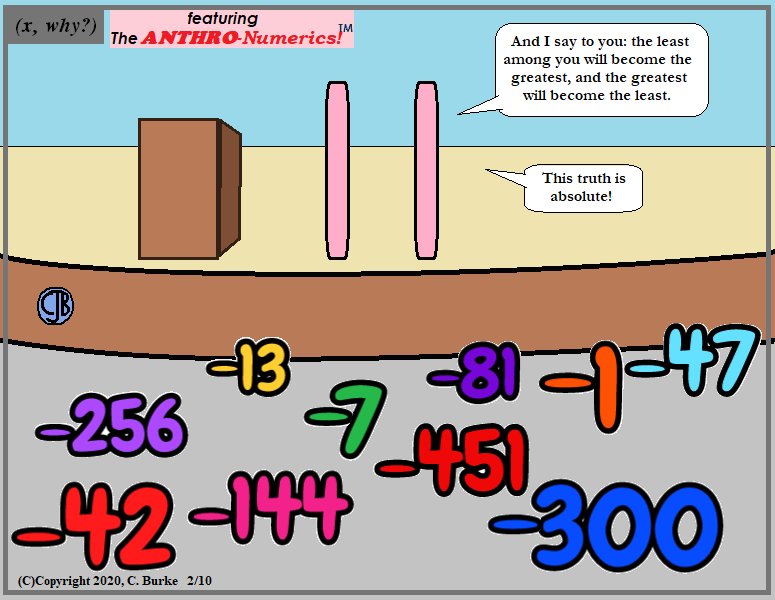(C)Copyright 2020, C. Burke. "AnthroNumerics" is a trademark of Christopher J. Burke and (x, why?).

I guess that goes for last being first and first being last when moved along the number line.

Come back often for more funny math and geeky comics.Friday, February 07, 2020

(x, why?) Mini: Cycling Through It

(Click on the comic if you can't see the full image.)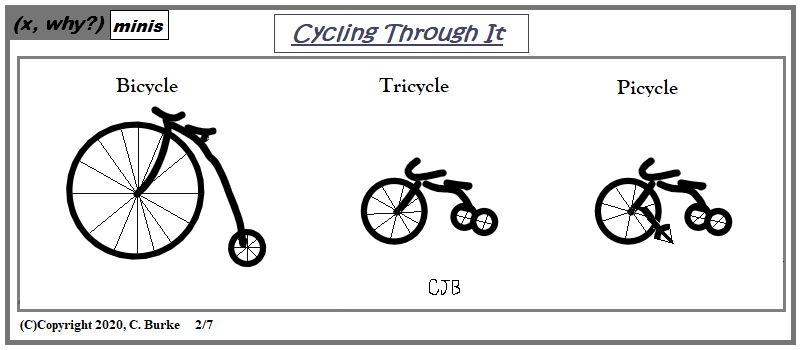(C)Copyright 2020, C. Burke. "AnthroNumerics" is a trademark of Christopher J. Burke and (x, why?).

Not sure what the .14 wheel does ...

Don't worry, I have another joke ready for March 14.

Come back often for more funny math and geeky comics.January 2020 Algebra Regents, Parts 3 & 4

The following are some of the multiple questions from the January 2020 New York State Common Core Algebra I Regents exam.

January 2020 Algebra I, Part III

Each correct answer is worth up to 4 credits. Partial credit can be given. Work must be shown or explained.

33. Michael threw a ball into the air from the top of a building. The height of the ball, in feet, is modeled by the equation h = -16t2 + 64t + 60, where t is the elapsed time, in seconds. Graph this equation on the set of axes below.

Determine the average rate of change, in feet per second, from where Michael released the ball to when the ball reached its maximum height.

Enter the equation into your graphing calculator and look at the table. You will see that there are points at (0,60), (1,108), (2,124), (3,108), (4,60) and (5,-20).
If you find the Zero, or just check a few numbers, you will see that the ball will hit the ground between 4.7 and 4.8 seconds.

To find the average rate of change from when he released the ball to its maximum height, find the slope of the line between (0,60) and (2,124)
(124 - 60)/(2 - 0) = 64/2 = 32 ft per second.

34. Graph the system of inequalities

-x + 2y - 4 < 0
3x + 4y + 4 > 0

Stephen says the point (0, 0) is a solution to this system. Determine if he is correct, and explain your reasoning.

First thing you probably want to do is to rewrite the inequalities in either Standard or Slope-Intercept form.

 -x + 2y - 4 < 0 -x + 2y < 4 2y < x + 4 y < 1/2 x + 2 3x + 4y + 4 > 0 3x + 4y > -4 4y > -3x - 4 y > -3/4 x - 1

Notice that the coefficients for y in each inequality are positive, so the inequality symbols will not flip when rewriting them.
The first inequality is less than, so it will have a broken line and be shaded below.
The second inequality is greater than or equal to, so it will have a solid line and be shaded above.

In the first inequality, the y-intercept is 2 and the slope is 1/2, which you can use to plot other points on this boundary line.
In the second inequality, the y-intercept is -1 and the slope is -3/4, which you can use to plot other points on the solid line.

After you shade both solutions, label the area on the right side, where the two solutions overlap, with a big "S". All the points in this section, including those on the solid line, but not those on the broken line, are solutions to the system of inequalities.

Your graph should look like the following:

Stephen is correct that (0,0) is a solution to the system because (0,0) is in the solution area for both inequalities.

35. The following table represents a sample of sale prices, in thousands of dollars, and number of new homes available at the price in 2017.

State the linear regression function, f(p), that estimates the number of new homes available at a specific sale price, p. Round all values to the nearest hundredth.

State the correlation coefficient of the data to the nearest hundredth. Explain what this means in the context of the problem.

Put the first row of numbers into List 1 (L1) in your calculator. Put the second row of numbers into List 2 (L2) in your calculator. Do a linear regression.

You should get, to the nearest hundredth, a=-.79, b=249.86 and r=-.95
The function, f(p) = -.79p + 249.86.

The correlation coefficient is -.95. This is a strong negative correlation. The higher the prices of the homes, the fewer homes are available.

36. The length of a rectangular sign is 6 inches more than half its width. The area of this sign is 432 square inches. Write an equation in one variable that could be used to find the number of inches in the dimensions of this sign.

Solve this equation algebraically to determine the dimensions of this sign, in inches.

The length is six inches more than half the width.
So L = 1/2 W + 6
The area is 432 square inches, so (L)(W)=432.
Combine them into one equation and you get (1/2W + 6)(W) = 432
or 1/2W2 + 6W = 432

To solve this equation, set the right side equal to 0 and factor.

1/2W2 + 6W = 432
1/2W2 + 6W - 432 = 0
W2 + 12W - 864 = 0

Factors of 864: 2 X 432, 3 X 288, 4 X 216, 6 X 144, 8 X 108, 9 X 96, 12 X 72, 16 X 52, 18 X 48, 24 X 36

(W - 24)(w + 36) = 0
W = 24 or W = -36

The Width is 24, so the length is 1/2 (24) + 6 = 18.

If you didn't want to work out the factors, you could have used the Quadratic Formula to find the same results. Or Completing the Square, which would have been the easiest in this case because you would have had to find the square root of 900, which is 30.

W2 + 12W - 864 = 0
W2 + 12W = 864
W2 + 12W + 36 = 900
(W + 6)2 = 900
W + 6 = SQRT(900) = + 30
W = 30 - 6 = 24 or W = -30 - 6 = -36 (reject the negative number)

January 2020 Algebra I, Part IV

A correct answer is worth up to 6 credits. Partial credit can be given. Work must be shown or explained.

37. Two families went to Rollercoaster World. The Brown family paid \$170 for 3 children and 2 adults. The Peckham family paid \$360 for 4 children and 6 adults.

If x is the price of a child's ticket in dollars and y is the price of an adult's ticket in dollars, write a system of equations that models this situation.

State the coordinates of the point of intersection.

Explain what each coordinate of the point of intersection means in the context of the problem.

Create a system of equations from the information that you were given.
3x + 2y = 170
4x + 6y = 360

If you can graph those in Standard Form by finding the y-intercept and the slope, GREAT! Otherwise, rewrite them in slope-intercept form, which will allow you to use your graphing calculator to get the table.

 3x + 2y = 170 2y = -3x + 170 y = -3/2 x + 85 4x + 6y = 360 6y = -4x + 360 y = -4/6 x + 60 y = -2/3 x + 60

Plot a point at (0, 85) and use the slope (-3/2) to plot the rest of that line.
Plot a point at (0, 60) and use the slope (-2/3) to plot the rest of that line.
Label the lines! Either write Brown family and Peckham family next to the corresponding line, or write the original equation next to the line. It is important that you state which line is which.

The solution to this system of equations is the point of intersection. The coordinates of the point of intersection are (30, 40).
In the context of this problem, it means that the price of children's tickets are \$30 and the price of adult tickets is \$40.

End of Exam

How did you do?

I also write Fiction!

Check out In A Flash 2020, by Christopher J. Burke for 20 great flash fiction stories, perfectly sized for your train rides.
Available in softcover or ebook at Amazon.

If you enjoy it, please consider leaving a rating or review on Amazon or on Good Reads.

Thank you.Thursday, February 06, 2020

What Does PEMDAS Stand For?

(Click on the comic if you can't see the full image.)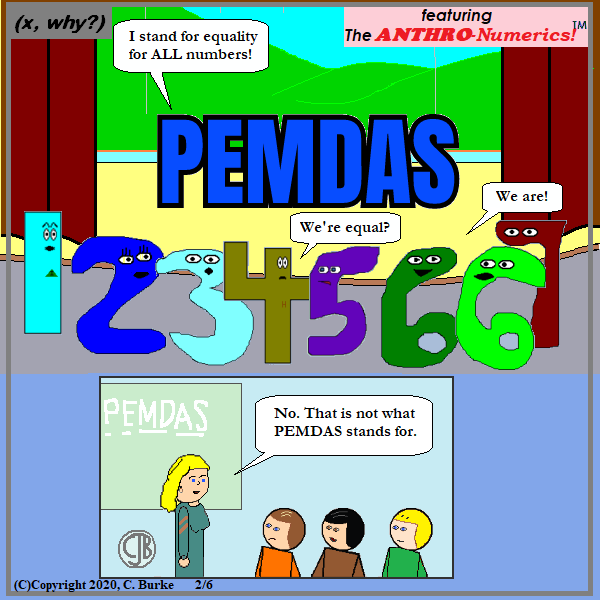(C)Copyright 2020, C. Burke. "AnthroNumerics" is a trademark of Christopher J. Burke and (x, why?).

Just keeping it Real.

Come back often for more funny math and geeky comics.Saturday, February 01, 2020

January 2020 Algebra Regents, Part 2

The following are some of the multiple questions from the January 2020 New York State Common Core Algebra I Regents exam.

January 2020 Algebra I, Part II

Each correct answer is worth up to 2 credits. Partial credit can be given. Work must be shown or explained.

25. Graph f(x) = -(sqrt(x)) + 1 on the set of axes below.

The function will have a domain of x > 0, meaning that the left side of the graph will be blank.
The y-intercept is 1. The negative in front of the radical means that line with curve down to the right, like half of a parabola that has been tipped onto its side.
Plot points using perfect squares for x -- 0, 1, and 4.
We already have (0, 1). The square root of 1 is 1; make it negative, it becomes -1; add 1, it becomes 0. Plot (1, 0). The square root of 4 is 2; make it negative, it becomes -2; add 1, it becomes -1. Plot (4, -1).
See image below.

26. Maria orders T-shirts for her volleyball camp. Adult-sized T-shirts cost \$6.25 each and youth-sized T-shirts cost \$4.50 each. Maria has \$550 to purchase both adult-sized and youth-sized T-shirts. If she purchases 45 youth-sized T-shirts, determine algebraically the maximum number of adult-sized T-shirts she can purchase.

Algebraically means write and equation with a variable, and solve for that variable. You must do this to get full credit because that's what you are instructed to do.

6.25x + 4.50(45) < 550
6.25x + 202.50 < 550
6.25x < 347.50
x < 55.6
The maximum number of shirts that Maria can order is 55.

27. A news report suggested that an adult should drink a minimum of 4 pints of water per day. Based on this report, determine the minimum amount of water an adult should drink in fluid ounces, per week.

Two conversions need to happen here: days to weeks, and pints to fluid ounces.
If you did not know that 1 pint = 16 fluid ounces, the reference table in the back of the booklet tells you that 1 pint = 2 cups, and 1 cup = 8 fluid ounces. Therefore 1 pint = (2)(8) = 16 fluid ounces.

4 pints / day * (7 days / week) * 16 (fluid ounces / pint)
= 4 pints / day * (7 days / week) * 16 (fluid ounces / pint)
= 4 * 7 * 16 (fluid ounces / week)
= 448 fluid ounces / week

You only needed to say 448. However, if you added units, you had to have them stated correctly.

28. Express (3x - 4)(x + 7) - (1/4)x2 as a trinomial in standard form.

Step one: multiply the binomials.
Step two: combine like terms.
Step three: rewrite in standard form, in you didn't do that already.

(3x - 4)(x + 7) - (1/4)x2
3x2 + 21x - 4x - 28 - (1/4)x2
2 3/4 x2 + 17x - 28

29. John was given the equation 4(2a + 3) = -3(a - 1) + 31 - 11a to solve. Some of the steps and their reasons have already been completed. State a property of numbers for each missing reason.

 4(2a + 3) = -3(a - 1) + 31 - 11a Given 8a + 12 = -3a + 3 + 31 - 11a __________________________ 8a + 12 = 34 - 14a Combining like terms 22a + 12 = 34 __________________________

The first blank is the Distributive Property. The second is Equally adding to both sides.
These are definitions: you either know these properties or you do not. You can't make up stuff or just write anything or "figure it out".

Note: I originally had "Zero Property of Addition" written in my answer, but that would not be correct as a step would need to be shown adding 14a to both sides, so that they could create a zero.
According to the model responses, just writing "Addition" would be enough.

30. State whether the product of SQRT(3) and SQRT(9) is rational or irrational. Explain.

The product of SQRT(3) * SQRT(9) = SQRT(27), which is equal to 3 SQRT(3), which is irrational because the product of a rational and an irrational number is always irrational.

You could have simplified SQRT(9) = 3 first, and skipped the SQRT(27) part.

31. Use the method of completing the square to determine the exact values of x for the equation x2 - 8x + 6 = 0.

If you are unsure of this, remember: you have the time. You can solve it another way, such as on your graphing calculator. Once you know the answer, that may make completing the square easier for you. If it doesn't, remember that you still get half credit for a correct answer found using an incorrect method.
Also, exact values means that they want you to use radicals for the irrational numbers. Do NOT use approximate decimal values.

x2 - 8x + 6 = 0, the middle term is -8, and half of -8 is -4. So (x - 4) will be in the final answer.
(-4)2 = 16, so we need to add 16 to both sides.
x2 - 8x + 16 + 6 = 16
x2 - 8x + 16 = 10, factor the trinomial into a perfect square.
(x - 4)2 = 10, now take the square root of each side of the equation.
x - 4 = +SQRT(10)
x = 4 + SQRT(10)

32. A formula for determining the finite sum, S, of an arithmetic sequence of numbers is

S = (n / 2) (a + b),

where n is the number of terms, a is the first term, and b is the last term. Express b in terms of a, S, and n.

The only things that are important in this question are the formula and which variable you have to solve for. The rest would only be important if there is a question about the sum of an arithmetic sequence later in the test.

To express b in terms of the other variables means isolating it on one side of the equation. That means using inverse operations.
I'll show each step, although you might combine the first two.

S = (n / 2) (a + b)
2 S = n (a + b)
(2S)/n = a + b
(2S)/n - a = b

End of Part II

How did you do?

I also write Fiction!

Check out In A Flash 2020, by Christopher J. Burke for 20 great flash fiction stories, perfectly sized for your train rides.
Available in softcover or ebook at Amazon.

If you enjoy it, please consider leaving a rating or review on Amazon or on Good Reads.

Thank you.